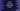# Swift tutorial : Recursive enumeration in Swift### Recursive enumeration in Swift : Explanation with example :

We know that a function is called recursive if the function itself is called from it. Similar to recursive function, in swift we have recursive enumeration. If any case in the enumeration contains one or more associated value as another instance of the Enumeration itself. For example, let’s take a look at the below example :

`````` enum RecursiveEnum {
indirect case firstCase(RecursiveEnum)
}``````

It is an recursive enumeration. Because, the case firstCase has associated value as same type of RecursiveEnum. One thing we should note that the keyword indirect should be used before the name of each case that will behave recursively. We can also use the indirect keyword before the name of the enum like below :

`````` indirect enum RecursiveEnum {
case firstCase(RecursiveEnum)
}``````

This is better than the previous version because we don’t need to write indirect before each case statement.To understand more on recursive enum, let me explain you with an example :

### Recursive Enum example :

Let’s consider the below enum :

`````` indirect enum Calculator{
case number(Int)
case subtract(Calculator, Calculator)
case multiply(Calculator, Calculator)
case divide(Calculator, Calculator)
}``````
• The name of the enum is Calculator
• It has four different cases .
• The case add, subtract, multiply and divide are of recursive type i.e. they will accept only values of type Calculator.

Now, to solve any expressions, we can write one function like below :

`````` func calculate(_  exp: Calculator) -> Int{
switch(exp){
case let .number(value):
return value
return calculate(first) + calculate(second)
case let .subtract(first , second):
return calculate(first) - calculate(second)
case let .multiply(first , second):
return calculate(first) * calculate(second)
case let .divide(first, second):
return calculate(first)/calculate(second)
}
}``````

This function will take one variable of type Calculator and find out the result recursively. For example, let’s take a look at the below example expressions :

``````let one = Calculator.number(1)
let two = Calculator.number(2)

print(calculate(Calculator.subtract(one,two)))
print(calculate(Calculator.multiply(one,two)))
print(calculate(Calculator.divide(two,one)))``````

It will print :

`````` 3
-1
2
2``````

Similarly, to solve one more complex problem like ( 2 + 3) * (5 - 2), we can use the below expression :

`````` let two = Calculator.number(1)
let three = Calculator.number(2)
let five = Calculator.number(5)